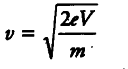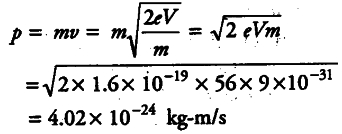# Calculate the momentum

Calculate the momentum.

Given, potential difference, V = 56 V
Use the formula for kinetic energy,
eV = 1/2 m\${{v}^{2}} = 2eV /m = {{v}^{2}}\$where, m is mass and v is velocity of electron. Momentum associated with accelerated electron,